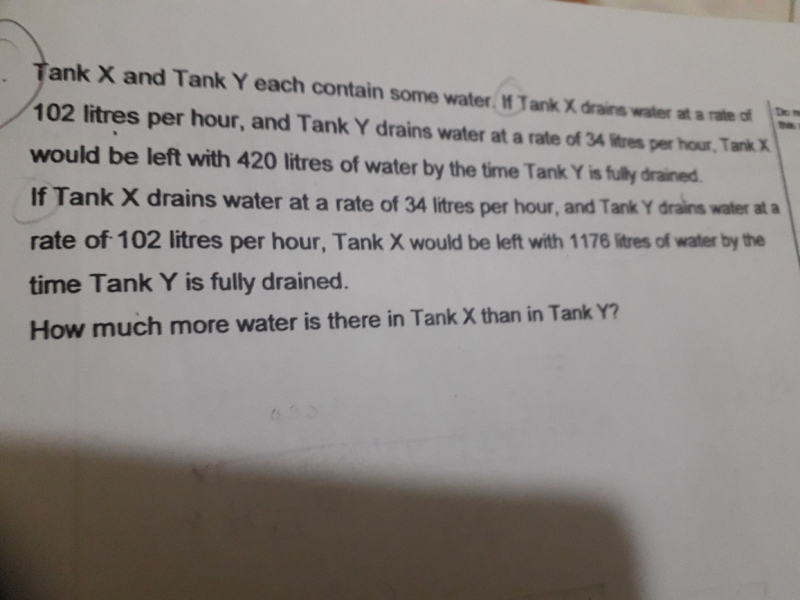# QuestionHow to solve

Step 1: Write down the draining ratio for both scenarios

Scenario 1:
X : Y
104 : 34
3 : 1

Scenario 2:
X : Y
34 : 104
1 : 3

Step 2: Make the ratio of Tank Y the same since it is a constant (completely drained in both scenarios)

Scenario 1:
X : Y
104 : 34
3 : 1
9 : 3

Scenario 2:
X : Y
34 : 104
1 : 3

Step 3: Identify that the difference in units for Tank X represents the difference in water left in Tank X for both scenarios

9 units – 1 unit = (1176 – 420)
8 units = 756

Step 4: Solve for both Tanks

1 unit = 94.5
3 units = 94.5 x 3 = 283.5 litres (water in Tank Y at first since it is completely drained)

From scenario 2:
94.5 + 1176 = 1270.5 litres (water in Tank X at first)

Step 5: Find difference in volume between both tanks

Difference in water volume:
1270.5 – 283.5 = 987 litres

0 Replies 0 Likes
 X Y Left in X (litres) Draining speed a (litres/hour) 102 34 420 Draining speed b (litres/hour) 34 102 1176

In speed a,  3Y+420 = X

In speed b, 1/3Y + 1176 = X

Hence, 3Y + 420  = 1/3 Y + 1176

9/3Y – 1/3Y =  1176 – 420

8/3Y = 756

Y = 756 x 3 ÷8

Y = 283.5 litres

X-Y = 3Y +420 – Y

X-Y = 2Y + 420

X-Y = 567 + 420

X-Y = 987 litres

0 Replies 0 Likes